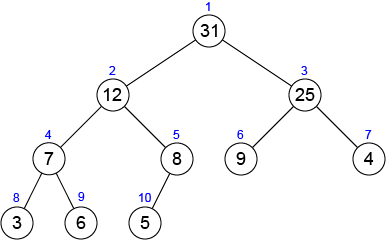Time Limit : sec, Memory Limit : KB

# Maximum Heap

A binary heap which satisfies max-heap property is called max-heap. In a max-heap, for every node $i$ other than the root, $A[i] \leq A[parent(i)]$, that is, the value of a node is at most the value of its parent. The largest element in a max-heap is stored at the root, and the subtree rooted at a node contains values no larger than that contained at the node itself.

Here is an example of a max-heap.Write a program which reads an array and constructs a max-heap from the array based on the following pseudo code.

$maxHeapify(A, i)$ move the value of $A[i]$ down to leaves to make a sub-tree of node $i$ a max-heap. Here, $H$ is the size of the heap.

1  maxHeapify(A, i)
2      l = left(i)
3      r = right(i)
4      // select the node which has the maximum value
5      if l ≤ H and A[l] > A[i]
6          largest = l
7      else
8          largest = i
9      if r ≤ H and A[r] > A[largest]
10         largest = r
11
12     if largest ≠ i　// value of children is larger than that of i
13         swap A[i] and A[largest]
14         maxHeapify(A, largest) // call recursively


The following procedure buildMaxHeap(A) makes $A$ a max-heap by performing maxHeapify in a bottom-up manner.

1 buildMaxHeap(A)
2    for i = H/2 downto 1
3        maxHeapify(A, i)


## Input

In the first line, an integer $H$ is given. In the second line, $H$ integers which represent elements in the binary heap are given in order of node id (from $1$ to $H$).

## Output

Print values of nodes in the max-heap in order of their id (from $1$ to $H$). Print a single space character before each value.

## Constraint

• $1 \leq H \leq 500,000$
• $-2,000,000,000 \leq$ value of a node $\leq 2,000,000,000$

## Sample Input 1

10
4 1 3 2 16 9 10 14 8 7


## Sample Output 1

 16 14 10 8 7 9 3 2 4 1


## Reference

Introduction to Algorithms, Thomas H. Cormen, Charles E. Leiserson, Ronald L. Rivest, and Clifford Stein. The MIT Press.